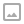This page looks best with JavaScript enabled

## Rotation Math Tricks - Using Unity's Quaternion Operators

·   ·  ☕ 2 min read

Unity provides a few operators that can make some common tasks with quaternions easier to accomplish. You can multiple a quaternion and vector to rotate the vector by the provided quaternion’s rotation or multiple two quaternions together in order to add the two rotations they represent together. These features make it easier to orient vectors in 3D space and can improve how you handle rotations (one common pattern I’ve seen that this can replace is the use Quaternion.Euler with a series of stored X, Y and Z rotations).

### Examples

If we wanted to rotate a forward facing vector by 45 degrees along the Y axis we might write something that looks like this:

 1 2 3  var originalVector = Vector3.forward; var rotation = Quaternion.Euler(0, 45, 0); var rotatedVector = rotation * originalVector; 

Note: multiplying a quaternion and vector requires that the quaternion be on the left hand side of the operation and the vector be on the right hand side. You will see an error otherwise. Use Quaternion * Vector not Vector * Quaternion.

To perform a 90 degree rotation around the X, Y and Z axis you might do this:

 1 2 3 4  var x = Quaternion.Euler(90, 0, 0); var y = Quaternion.Euler(0, 90, 0); var z = Quaternion.Euler(0, 0, 90); var rotation = x * y * z; // Add the three rotations above 

Unity has provided additional examples and documentation for Quaternion multiplication operations here: https://docs.unity3d.com/ScriptReference/Quaternion-operator_multiply.html

Join the World of Zero Discord Community: https://discord.gg/hU5Kq2uWRITTEN BY
Sam Wronski
Maker of things, currently helping build cloud things @ Google. World of Zero is a personal project disconnected from my professional work. Lets make something awesome together!